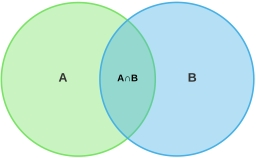# School student

The probability that a school student has a skateboard is 0.34, the probability that he has a bicycle is 0.81 and the probability that he has a skateboard and a bicycle is 0.22. What is the probability that a randomly selected student has a skateboard or bicycle?Did you find an error or inaccuracy? Feel free to write us. Thank you!Tips to related online calculators
Would you like to compute count of combinations?